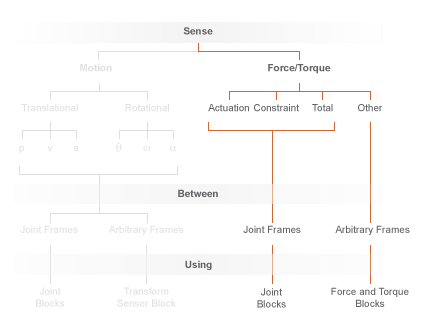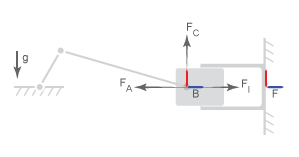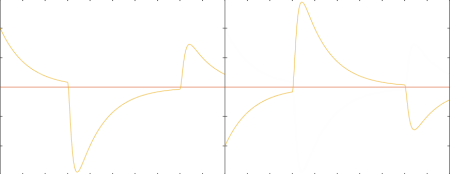## Force and Torque Sensing

Blocks with force and torque sensing appear in two Simscape™ Multibody™ libraries:

• Forces and Torques — Measure the magnitude of certain forces not explicitly provided by input. Blocks with force sensing include Inverse Square Law Force and Spring and Damper Force. Each block can measure only the magnitude of its own force.

• Joints — Measure various forces and torques acting directly at a joint. All joint blocks provide force and torque sensing. However, the specific force and torque types that you can measure vary from joint to joint. Force and torque sensing is available strictly between the bodies the joint connects.

Force and Torque Sensing in Simscape Multibody### Joint Forces and Torques You can Measure

Forces and torques that you can measure at a joint fall into two categories:

• Joint primitive forces and torques. Each such force or torque is individually computed for a given joint primitive. Joint actuator forces and torques belong to this category.

• Composite forces and torques. Each such force or torque is computed in aggregate for an entire joint. Constraint and total forces and torques belong to this category.

The table summarizes the different joint forces and torques.

Force/Torque TypeActs OnMeasures
ActuatorIndividual joint primitivesForce or torque driving an individual joint primitive. The measured force or torque can be provided by input or it can be automatically computed based on joint motion inputs in a model.
ConstraintEntire jointsAggregate constraint force or torque opposing motion normal to the joint degrees of freedom. By definition, these forces and torques act orthogonally to the joint primitive axes.
TotalEntire jointsNet sum of all forces or torques acting between the joint port frames. These include actuator, internal, and constraint forces and torques.

The figure shows a basic example of these forces acting on a crank-slider piston.In the figure:

• FA is the actuator force, which drives the piston toward the crank link.

• FI is the internal spring and damper force, which resists motion of the piston with respect to the chamber.

• FC is the constraint force, which opposes the effect of gravity on the piston, preventing it from falling.

The total force equals the net sum of FA, FI, and FC.

### Force and Torque Measurement Direction

In accordance with Newton’s third law of motion, a force or torque acting between two joint port frames accompanies an equal and opposite force or torque. If the base port frame of a Prismatic Joint block exerts a force on the follower port frame, then the follower port frame exerts an equal force on the base frame. When sensing composite forces and torques in joint blocks, you can specify which of the two to measure:

• Follower on base — Measure the force or torque that the follower port frame exerts on the base port frame.

• Base on follower — Measure the force or torque that the base port frame exerts on the follower port frame.

The figure shows the effect of reversing the measurement direction. Reversing this direction changes the measurement sign.